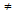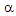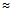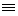# Electronics - Combinational Logic Circuits

### Exercise :: Combinational Logic Circuits - General Questions

6.

When two or more inputs are active simultaneously, the process is called:

 A. first-in, first-out processing B. priority encoding C. ripple blanking D. priority decoding

Answer: Option B

Explanation:

No answer description available for this question. Let us discuss.

7.

Which type of decoder will select one of sixteen outputs, depending on the 4-bit binary input value?

 A. hexadecimal B. dual octal outputs C. binary-to-hexadecimal D. hexadecimal-to-binary

Answer: Option A

Explanation:

No answer description available for this question. Let us discuss.

8.

A magnitude comparator determines:

 A. AB and if AB or A >> B B. AB and if A > B or A < b C. A = B and if A > B or A < b D. AB and if A < b or a > B

Answer: Option C

Explanation:

No answer description available for this question. Let us discuss.

9.

A circuit that responds to a specific set of signals to produce a related digital signal output is called a(n):

 A. BCD matrix B. display driver C. encoder D. decoder

Answer: Option C

Explanation:

No answer description available for this question. Let us discuss.

10.

Which digital system translates coded characters into a more intelligible form?

 A. encoder B. display C. counter D. decoder

Answer: Option D

Explanation:

No answer description available for this question. Let us discuss.

#### Current Affairs 2021

Interview Questions and Answers